University of California, Berkeley EECS Dept, CS Division Jordan Smith SLIDE: Scene Language for Interactive Dynamic Environments Prof. Carlo H. Séquin

 Home Goals Publications People Gallery Assignments Distributions

# SLIDE 2D Linear Algebra Notes

## Vector Spaces and Affine Spaces

A vector space is a set of vectors which have a vector addition (u+v) and a scalar multiplication (cv). The vector spaces we will use most commonly in graphics are R2 and R3, the 2D and 3D real coordinate systems.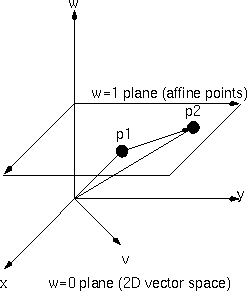For our purposes, an affine space a vector space offset from the origin.

We can represent points and vectors in an affine space by adding an extra coordinate. For points, this coordinate will be 1, for vectors it will be 0. So, in 2D the point, p at (1,1) will be written p=(1,1,1), but the vector v at (1,1) will be written v=(1,1,0).

You can visualize the 2D affine space as the w=1 plane in a 3-dimensional (x,y,w) space.

You can subtract points to get vectors (p1-p2=u), and you can add vectors to points to get other points (p1+u=p2), but you cannot add two points together in an affine space. (p1+p2 is outside the affine plane),

## Transformations in 2D

Type Column Vector Matrix Row Vector Matrix Properties
Rotation
`rotate (0 0 1) (a)`
 cos(a) -sin(a) 0 0 sin(a) cos(a) 0 0 0 0 1 0 0 0 0 1
 cos(a) sin(a) 0 0 -sin(a) cos(a) 0 0 0 0 1 0 0 0 0 1
Rigid Body
Orthogonal
Scale

`scale (sx sy 1)`
 sx 0 0 0 0 sy 0 0 0 0 1 0 0 0 0 1
 sx 0 0 0 0 sy 0 0 0 0 1 0 0 0 0 1
Non-Rigid (does not preserve length/volume)
Translation

`translate (tx ty 0)`
 1 0 0 tx 0 1 0 ty 0 0 1 0 0 0 0 1
 1 0 0 0 0 1 0 0 0 0 1 0 tx ty 0 1
Rigid Body
Orthogonal Submatrix

### Translation

 x' = x + tx y' = y + ty Translation can be written as a shear in an affine space.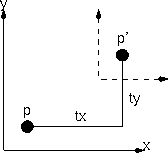### Rotation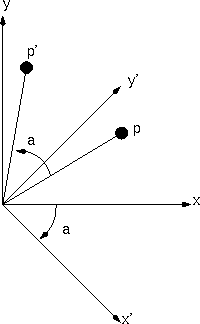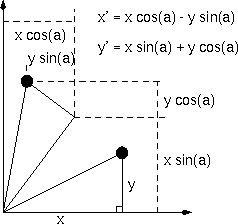Rotation can be thought of as representing a rotated coordinate system's basis vectors in a current coordinate system. Based on this mental approach, a rotation can be thought of as a change of basis matrix. Usually this change of basis is done with unit length basis vectors, in which case the rotation matrix is then orthogonal.

In the figure on the right, the point p is rotated by an angle a to the point p'. You could think of the same transformation as rotating the x-axis to x' and the y-axis to y'. Then the point p is in the correct rotated place with respect to x' and y'.

### Scale

 Uniform scale: p'=sp Non-uniform scale: x' = sxx y' = syy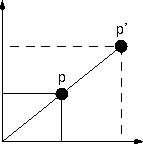### View Port

World Window = ( (xmin, ymin), (xmax, ymax) )
View Port = ( (umin, vmin), (umax, vmax) )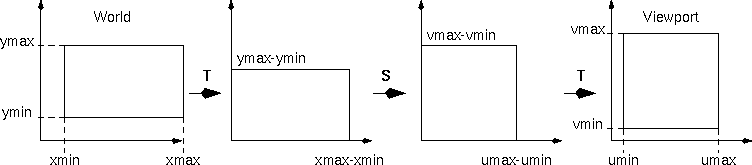MVP,W = T(umin, vmin) * S( (umax - umin)/(xmax - xmin), (vmax - vmin)/(ymax - ymin) ) * T(-xmin, -ymin)

## Matrix Properties

Transpose
(QR)T = RTQT
Inverse
Q Q-1 = I
(QR)-1 = R-1Q-1
Symmetric
Q = QT
Orthogonal
(QQT) = I
QT = Q-1
All of the columns of an orthogonal matrix are orthonormal. This means that
• Each column is a vector with length 1.
• Any two different columns are orthogonal (perpendicular) to each other.
In other words, for Q = qi j with rows Ri = qi * and columns Cj = q* j, then for all i and j
• i == j: CiTCj = 1
• i != j: CiTCj = 0